# Formula Use Cases

The Formula Column is one of the more popular ways to manipulate data on monday.com. From simple mathematical calculations to more complicated formulas, by utilizing our library of available functions, the Formula Column can help you simplify complex problems.

Before we dive into this article, in the below board, you will find some of our most common use cases for the Formula column:

Feel free to simply copy and paste the formula provided in the 'Formula' column directly onto your own board for you to use!Tip: To access our Formula Use Cases board, you can also click right here. 🙏

Now, let's further explore some of the use cases that we've collected. Below you'll find some of the most common ways to use the Formula Column ⬇️

# Adding or subtracting days from a date

In this example, we want to add 15 days to each date from the column "Start Date":

```ADD_DAYS({Start Date},15) ```

If we wanted to subtract 15 days instead, we would use the function SUBTRACT_DAYS() in place of ADD_DAYS().

```SUBTRACT_DAYS({Start Date},15) ```

Both of the above formulas will show you an unformatted result that may look a little clunky. This is why we recommend using the following formula. The result is formatted and will look cleaner on your board:

`FORMAT_DATE(ADD_DAYS({Start Date},15))`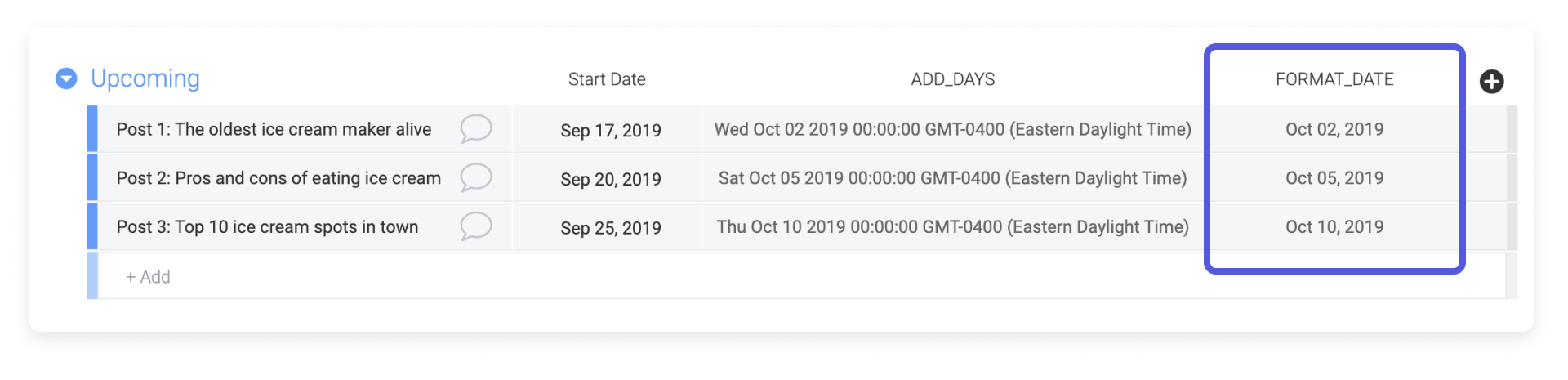If you want to remove the year and simply see the month and day, you can further customize your formula by using the LEFT() function. In the following formula, the LEFT() function takes the output of the FORMAT_DATE() function and only outputs 6 characters from the left:

```LEFT(FORMAT_DATE(ADD_DAYS({Start Date},15)),6) ```

If we change the 6 in the formula, we change the number of characters that the formula outputs. The results of this formula can be seen in the "LEFT" column: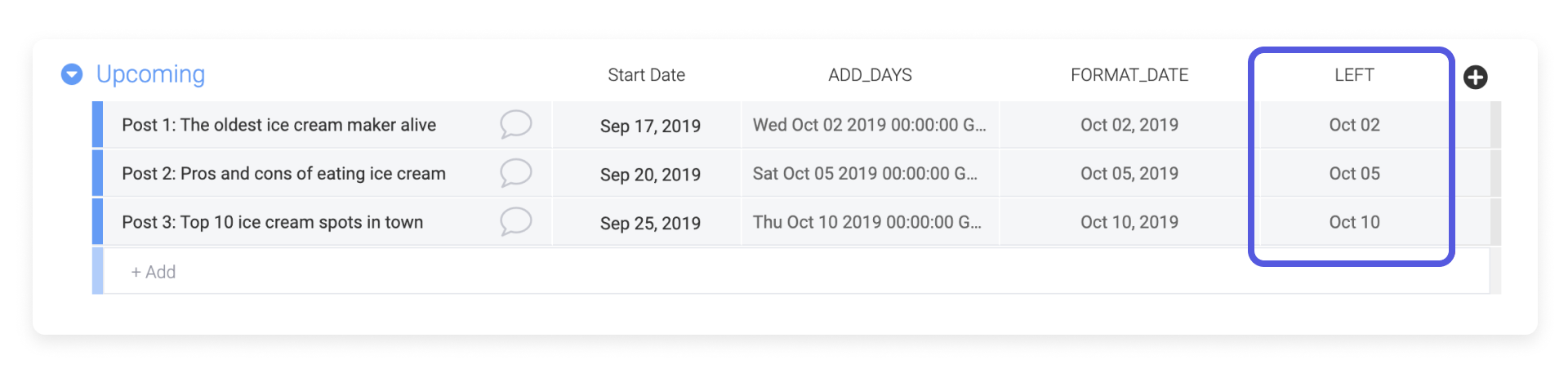# Calculating days between a date and today

One really useful and dynamic function is TODAY(). Using this with the DAYS() function, you can calculate the number of days until the due date (or past the due date). When using TODAY(), you don't need to include anything within the parenthesis.

We've also encapsulated the output of the DAYS() function within the ROUND() function. In this case, we're indicating that we want to round the output with 0 decimal places.

`ROUND(DAYS({Due Date},TODAY()),0)`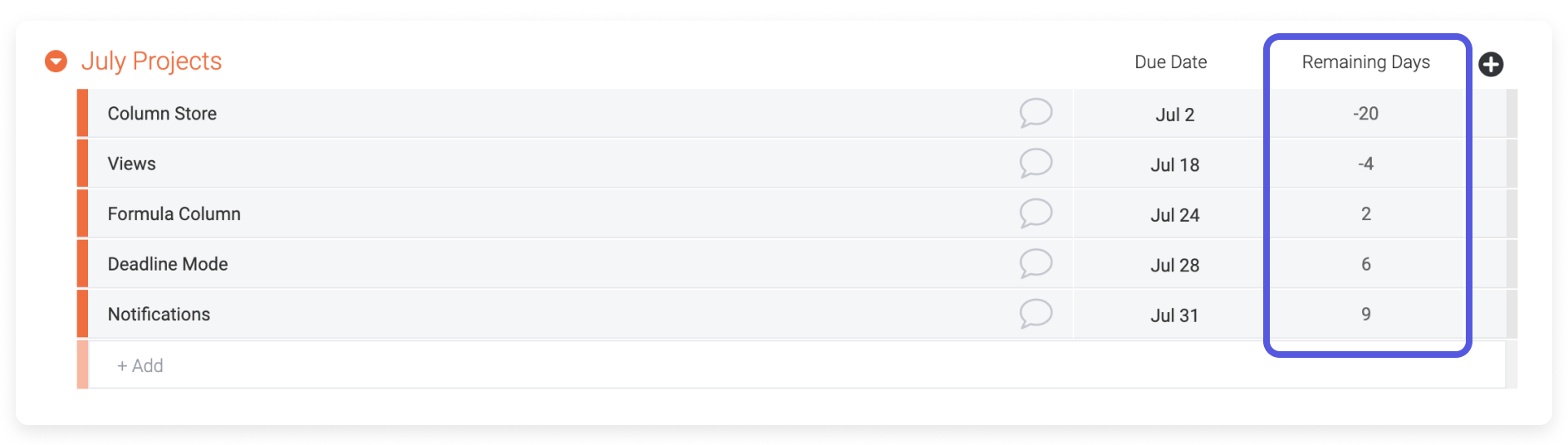# Determining the number of working days between two dates

If you're using a board to keep track of employee vacation requests, a formula can be useful when calculating the number of working days the employee will need. The following function, WORKDAYS(), will return the number of working days between two dates. Working days are defined, according to your account settings, as Monday to Friday or Sunday to Thursday. To learn more about this account setting, check out this article.

`WORKDAYS({To},{From})`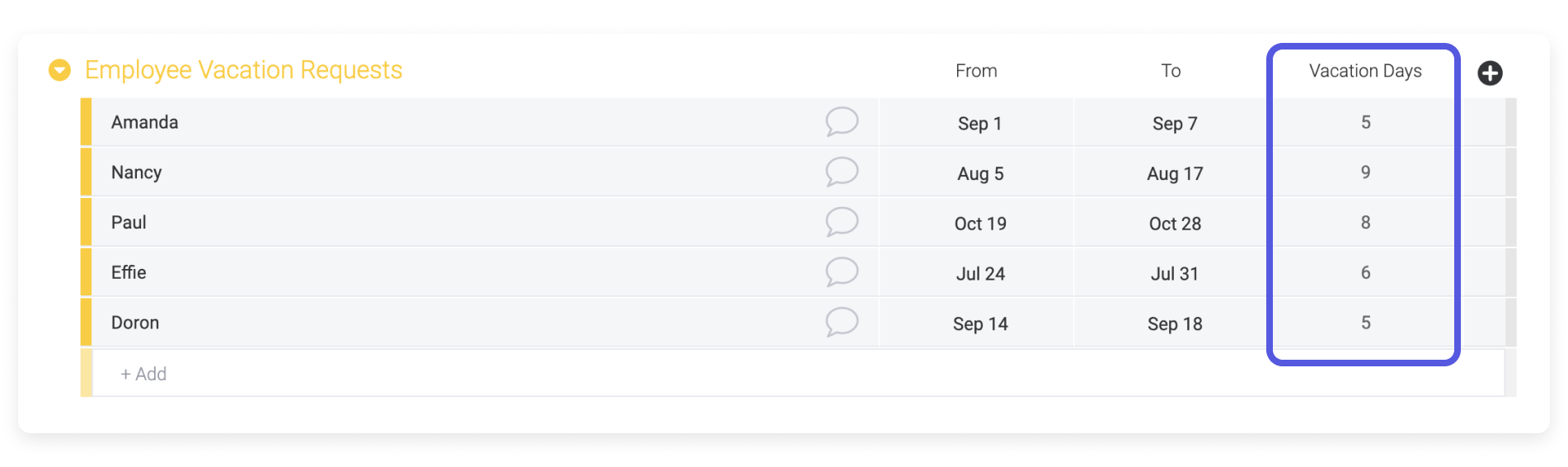# Calculating billable total using the time tracking column

When using the Time Tracking Column with the Formula Column, you can choose whether you want to pull in seconds, minutes, or hours. For the following formula, we're using the "Billable" column's hours. We're also using the ROUND() function again to clean up our decimals. The number 2 in this formula signifies two decimal places.

```ROUND(MULTIPLY({Billable#Hours},{Hourly Rate}),2) ```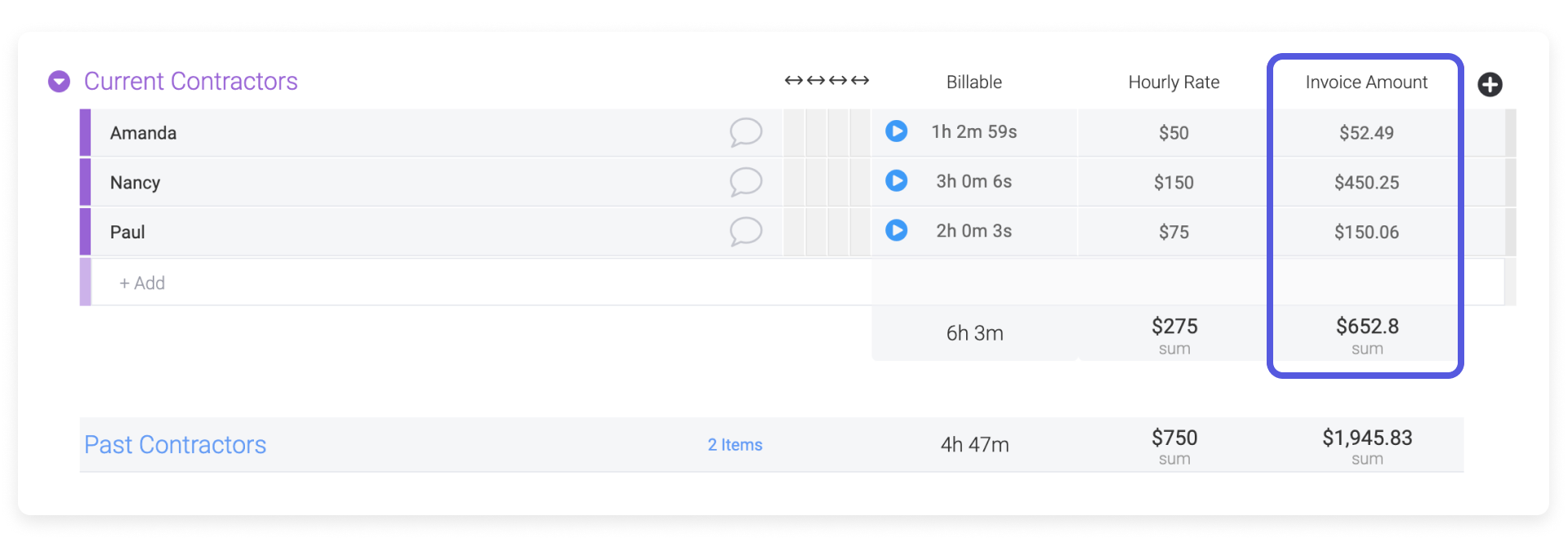# Totalling hours worked excluding breaks

In this example, I'd like to calculate how long my contractors have worked. This should not include their unpaid break time. Using four Hour Columns, I can create a formula to calculate this.

`IF(HOURS_DIFF({Break End}, {Break Start}) > "0", HOURS_DIFF(HOURS_DIFF({End}, {Start}), HOURS_DIFF({Break End}, {Break Start})), HOURS_DIFF({End}, {Start}))`

This formula says that if the break is greater than 0, calculate the total hours worked minus the break. If the break is not greater than 0, calculate the total hours worked.

Here's how the formula works: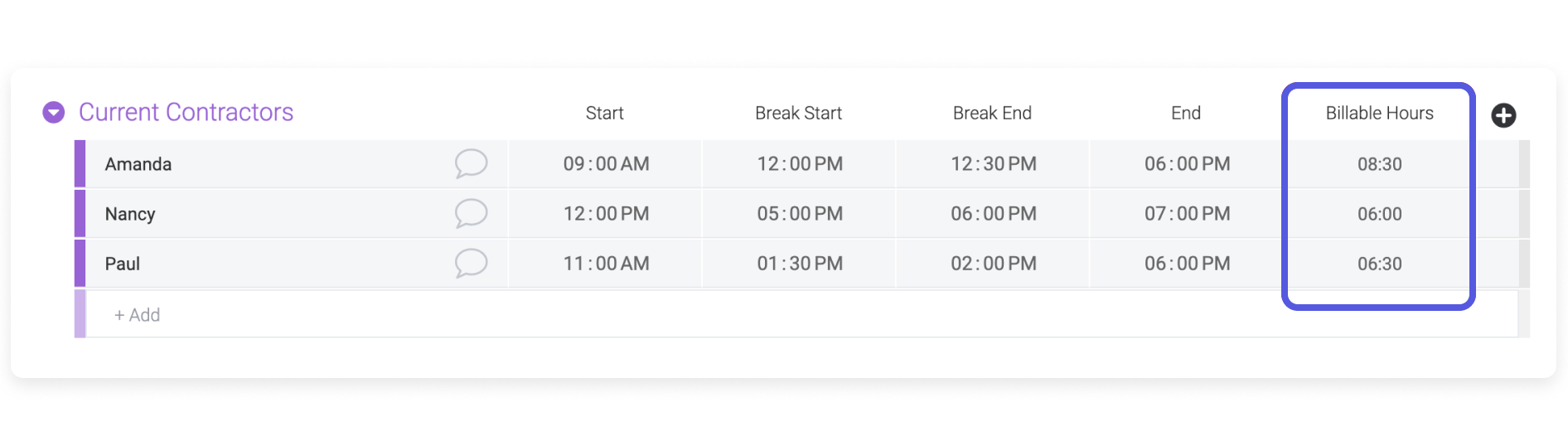# Calculating the change between two values

In this board, we're looking at the total sales per month for four employees. To calculate the change between the results of January and February as a percentage, you would use the following formula:

`MULTIPLY(DIVIDE(MINUS({February Sales},{January Sales}),{January Sales}),100)`

This formula can also be written as:

`((({February Sales}-{January Sales})/{January Sales})*100)`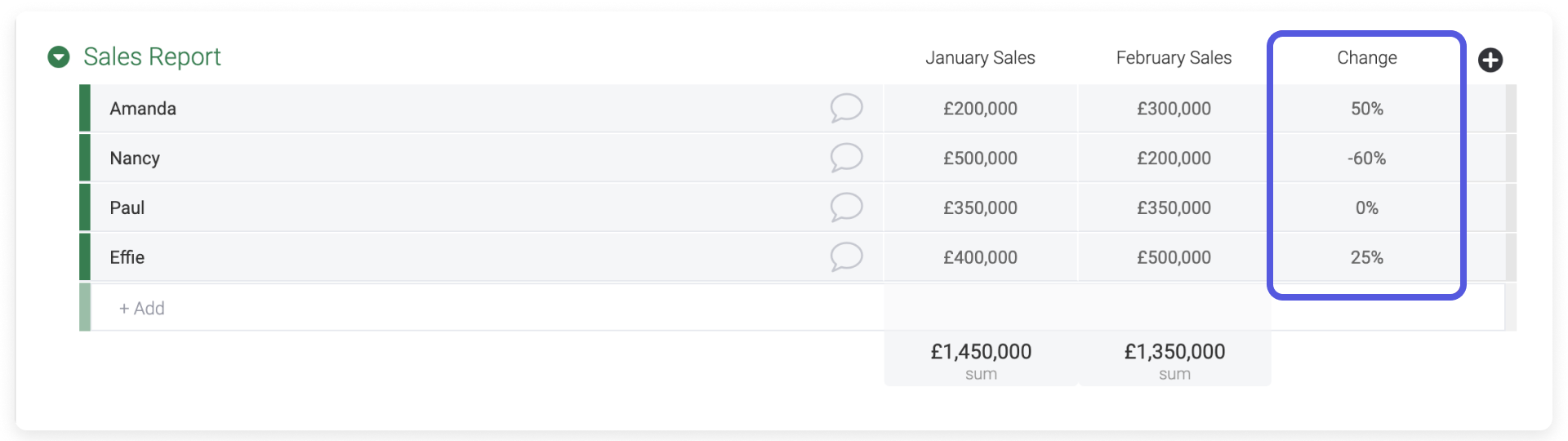# Outputting a value based on two conditions

Now we want to calculate each employee's bonus. An employee will receive a bonus only if the "Total Sales" are higher than \$350,000 and if the number of deals in the "Deals" column is higher than 12:

`IF(AND({Total Sales}>350000,{Deals}>12),250,0)`

The AND() function checks whether the two conditions are true. Based on the result, the IF() statement tells the formula column which value to return.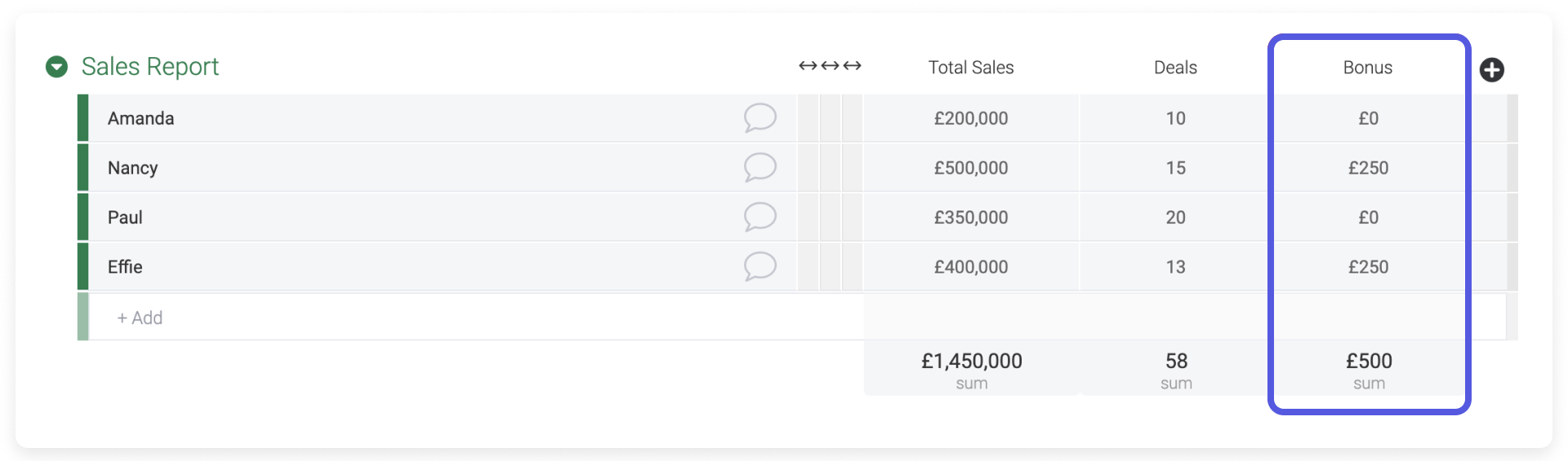# Using status labels to assign commission rates

In this example, let's say I manage a sales team with varying commission rates per salesperson.  You can use the labels from the status column to indicate a specific rate within your formula:

`IF({Rate}="Rate 1",25,IF({Rate}="Rate 2",20,IF({Rate}="Rate 3",15,IF({Rate}="Rate 4",10,IF({Rate}="Rate 5",5)))))`

The "Commission %" column is showing the relevant rate based on the status label selected.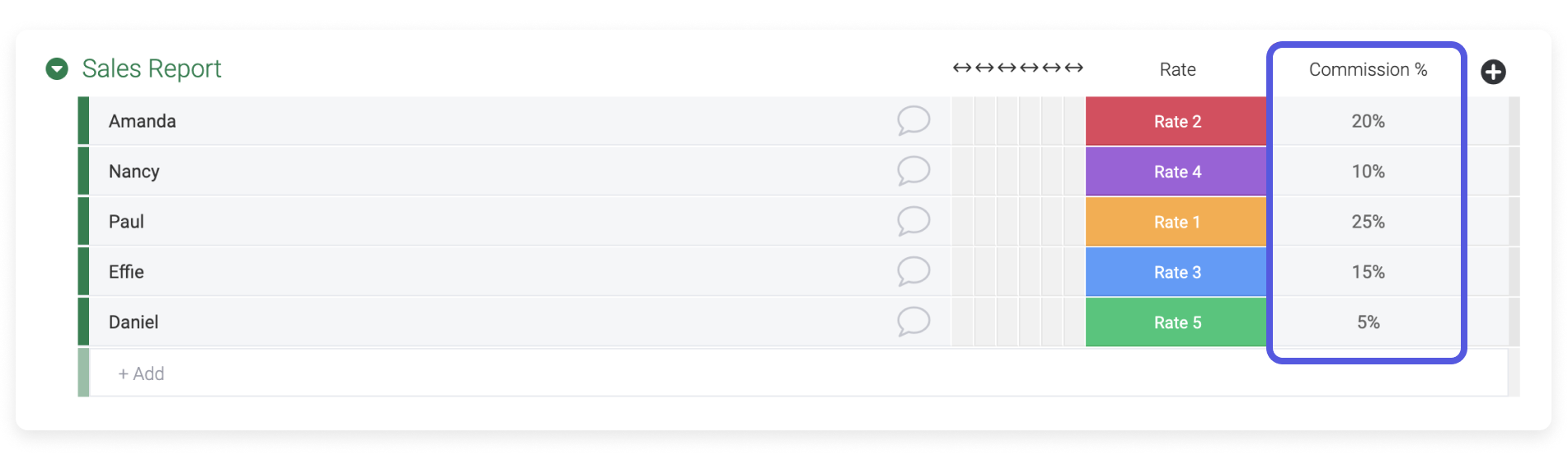You can take this formula a step further and calculate commission based on the rate and the "Total Sales" column by incorporating the MULTIPLY() function. Just keep in mind that if you use a decimal in a formula, you must write 0.25 rather than .25 to avoid an illegal formula error.

```IF({Rate}="Rate 1",MULTIPLY(0.25,{Total Sales}),IF({Rate}="Rate 2",MULTIPLY(0.20,{Total Sales}),IF({Rate}="Rate 3",MULTIPLY(0.15,{Total Sales}),IF({Rate}="Rate 4",MULTIPLY(0.10,{Total Sales}),IF({Rate}="Rate 5",MULTIPLY(0.05,{Total Sales})))))) ```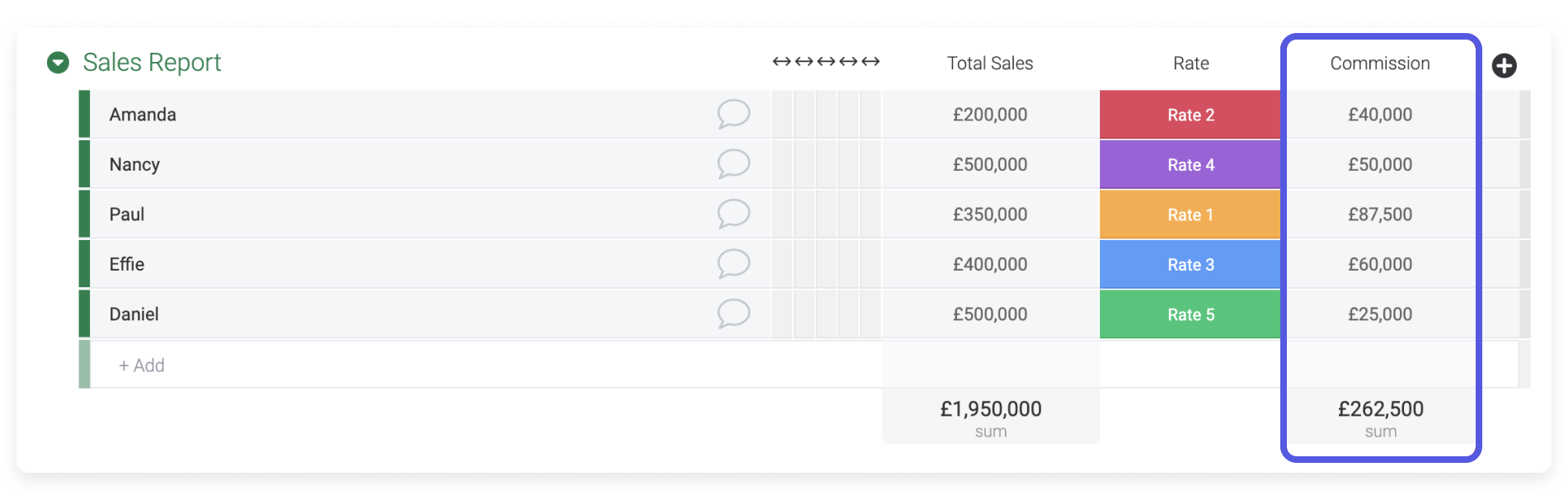# Comparing a dynamic value to a static value

If you're using a board to keep track of your budget, this formula might come in handy. In this example, the travel budget for each employee is \$6,500. We want to find out if the total amount spent on each employee is within the budget or over it. To do this, we will compare the SUM() of the values in four columns to the budget with an IF() statement.

`IF(SUM({Flight},{Hotel},{Insurance},{Expenses})>6500, "Over Budget","Good")  `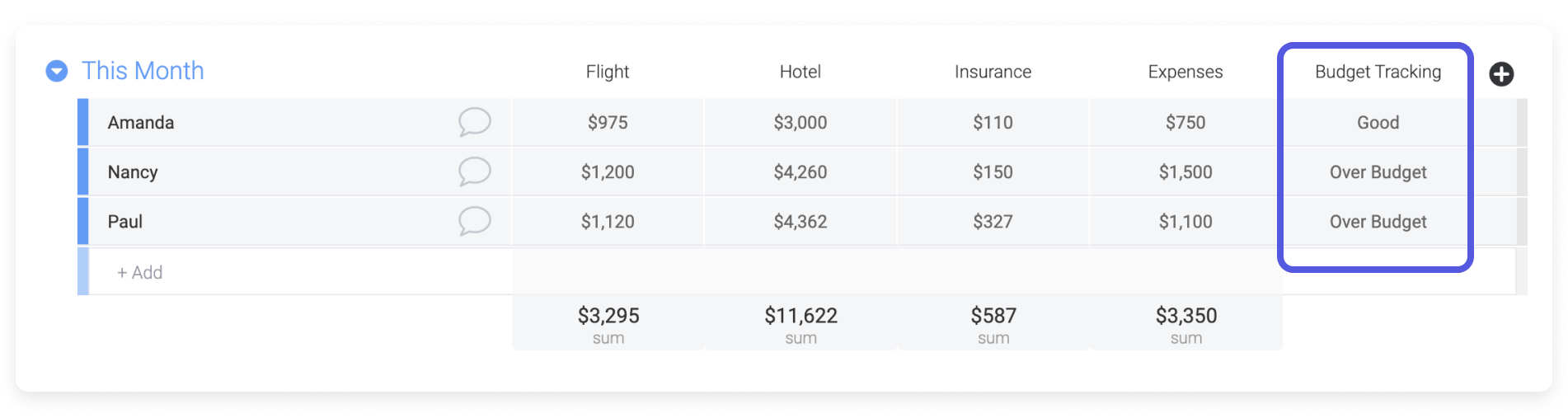# Tracking inventory

You can easily manage your inventory with monday.com. This example explains how to calculate your current available stock and how much inventory was sold (as a percentage).

For the "In Stock" column:

`MINUS(MINUS({Starting Inventory},{Reserved}),{Sold})`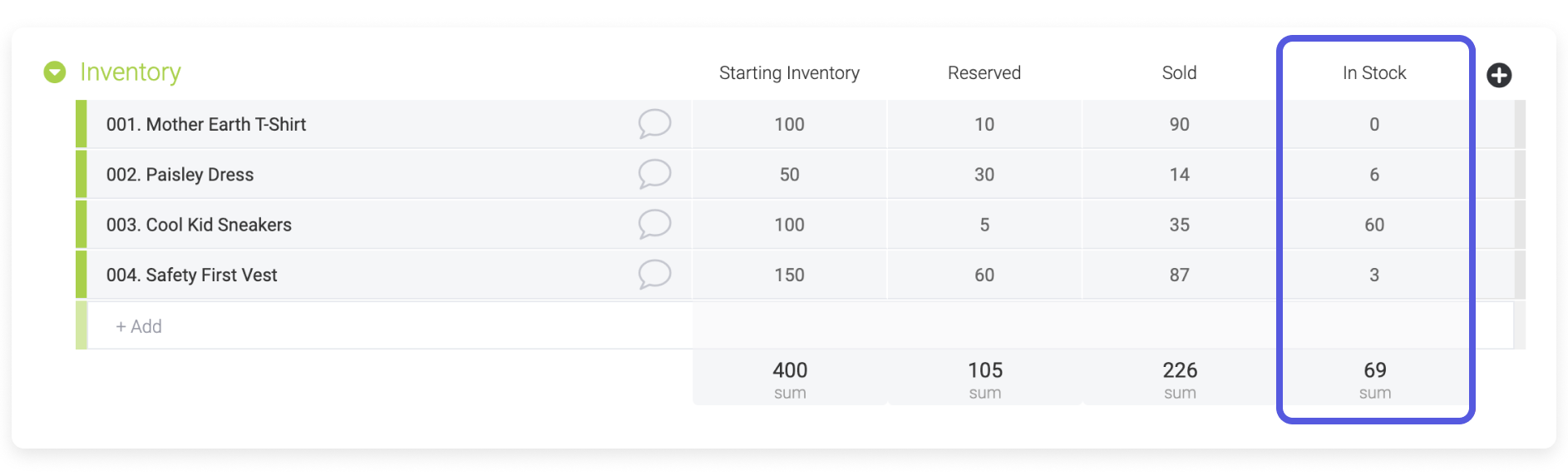For the "% Sold" column:

`ROUND(MULTIPLY(DIVIDE({Sold},{Starting Inventory}),100),2)`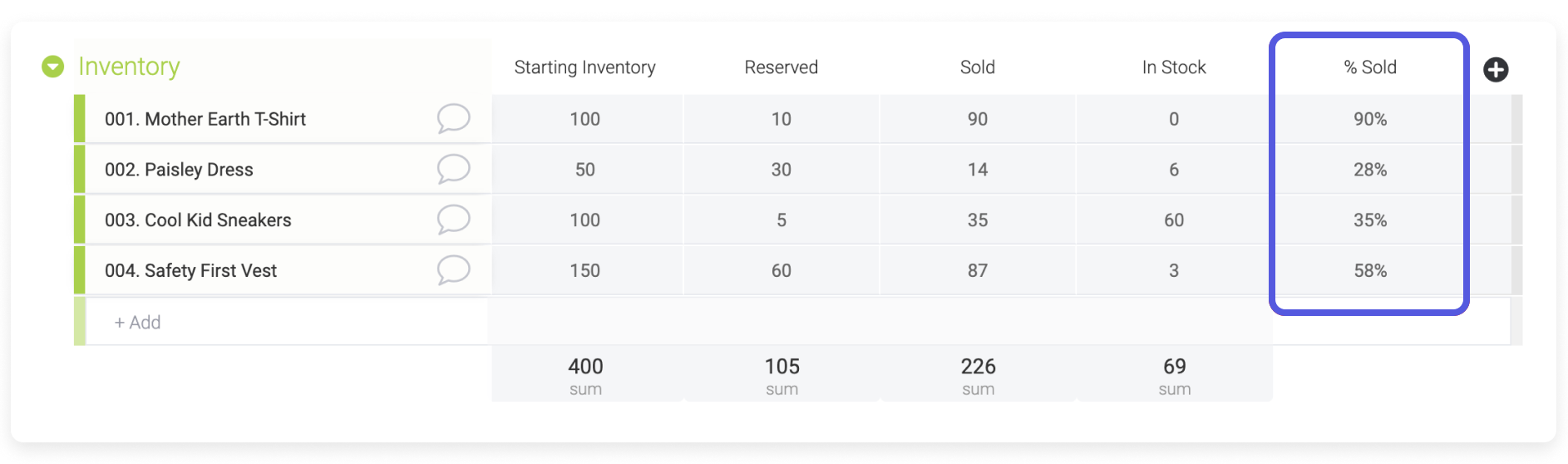# Formatting a formula

By using the TEXT() function, you can format your results exactly the way you want. Let's take an easy example in the first line of the following board. We want to multiply 100 by 25, and we want the output to show like this: \$2,500.00.

`TEXT(MULTIPLY({Starting Inventory},{Cost}),"\$#,##.00")`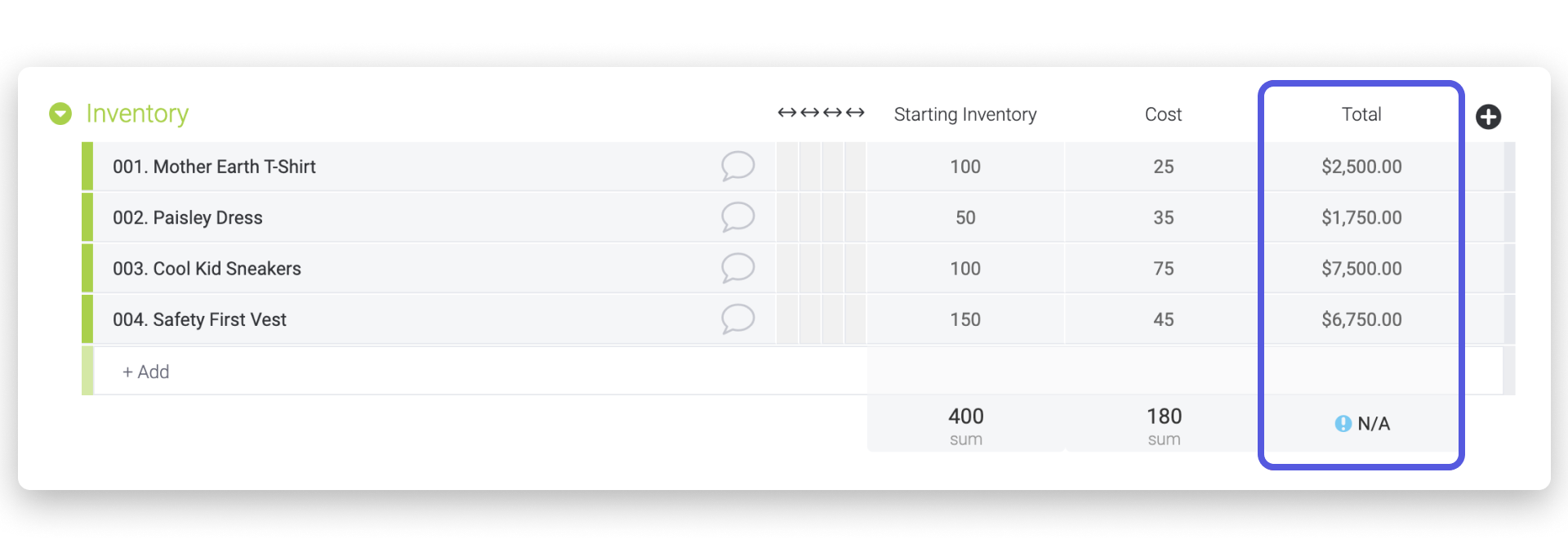In the last part of the formula, each # represents a number. We added .00 to the end because we want the output to end with two zeros, but you can replace this with .## if you want your output to end with calculated numbers. The \$ places the symbol in front of the number.

Keep in mind that if you format a number using the TEXT() function, the formula column will read the number as text rather than a number. This means that your column summary will show "N/A" (as above) instead of the sum/average/etc. of the numbers shown in your formula column.

If you still have questions, we're here for you! Check out the Community FAQ forum on formulas, or you can reach out to our team by using our contact form. We're available 24/7 and happy to help!

Have more questions? Submit a request